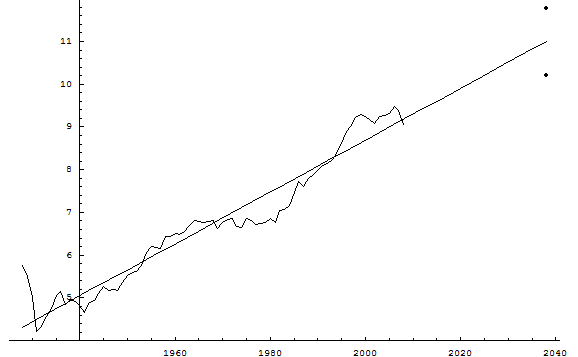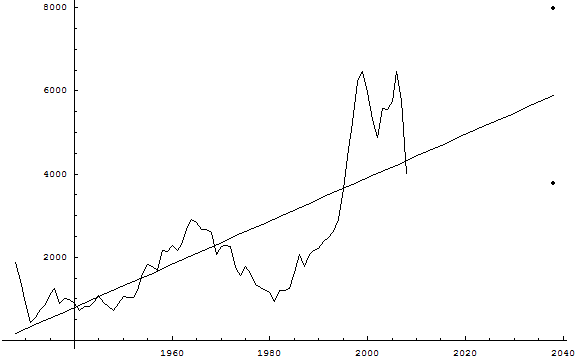Are you fortunate to be a young person planning to retire in 30 years? All financial advisers will tell you to invest in the stock market now and enjoy wealth when you retire. Here is a simple and rough estimate of your future fortune.

Consider the Dow-Jones Industrial Index data from 1928-2008 (annual averages), and put them on the logarithmic scale (see Figure 1). Perform linear regression on these data and find the 95% confidence interval in 2038. See the regression line and the endpoints of the confidence interval in Figure 1.

If you invest 10,000 dollars now, you will have between 27,000 dollars and 131,000 dollars in 2038. The return on your investment will be between 170% and 1,200%, with probability 95%.Figure 1

The naysayer version - free advice from 100% certified skeptical financial adviser.

Consider the Dow-Jones Industrial Index data from 1928-2008 (annual averages), and express them in constant dollars using the Consumer Price Index (see Figure 2). Perform linear regression on these data and find the 95% confidence interval in 2038. See the regression line and the endpoints of the confidence interval in Figure 2.

If you invest 4,000 dollars now, you will have between 3,800 dollars and 8,000 dollars in 2038. The return on your investment, in real terms, will be between 5% loss and 100% gain, with probability 95%.Figure 2

The predictions were made in November 2009.